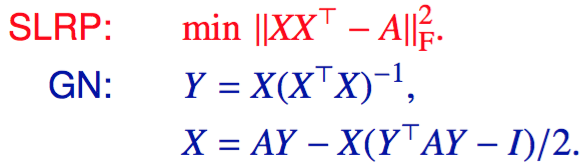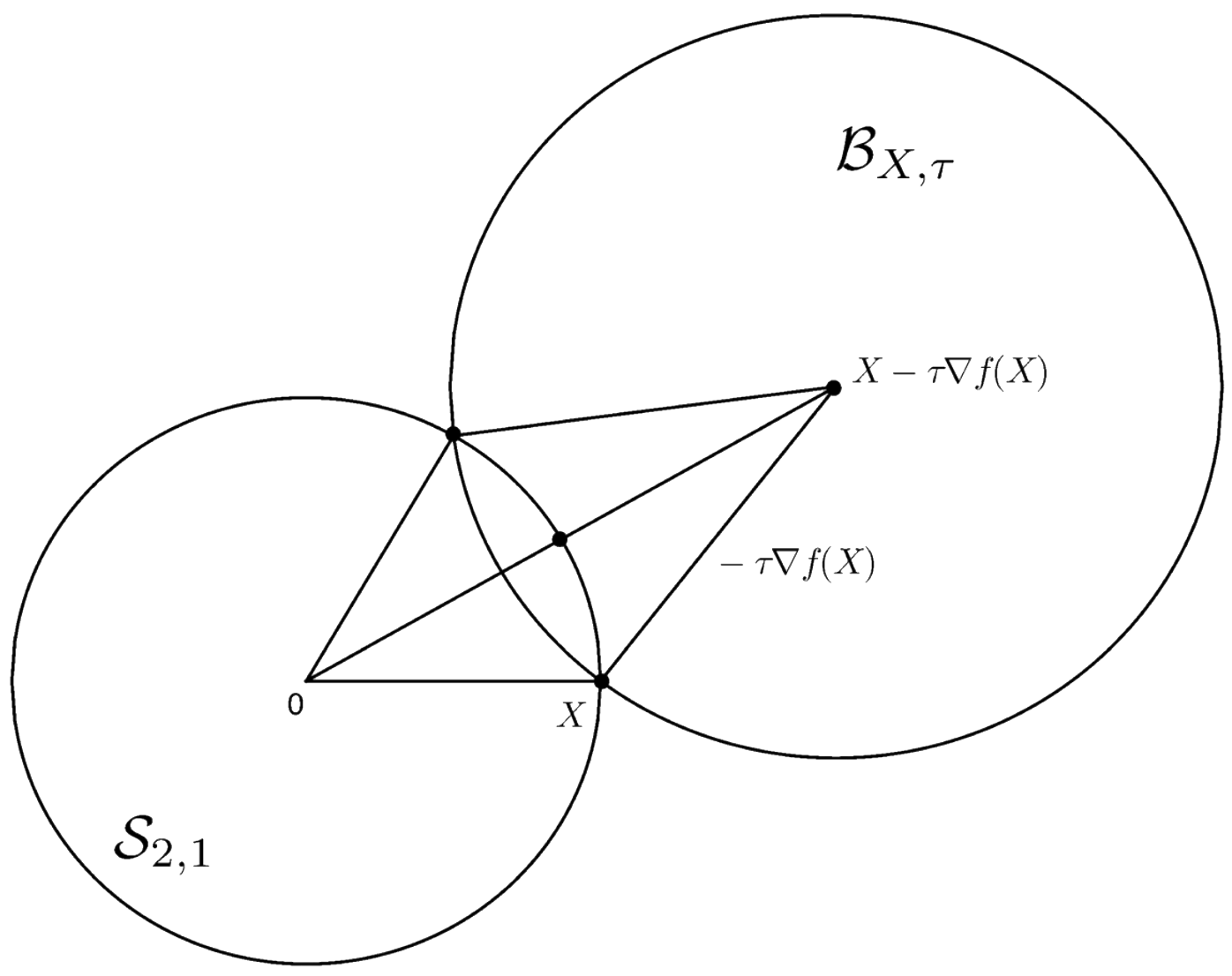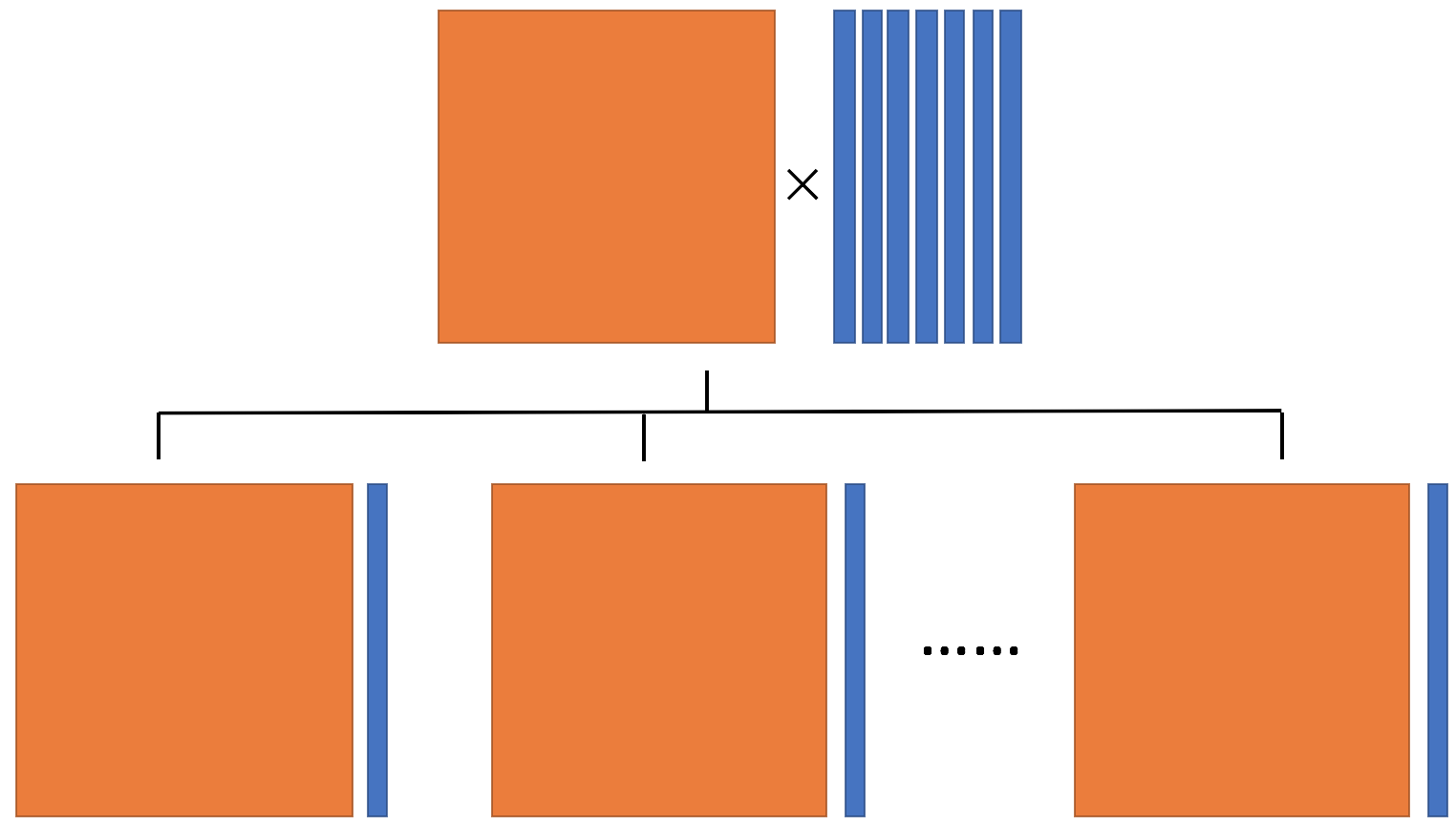# Solvers

## Dominant Eigenpair Computation

LMSVD is an adaptive Krylov subspace algorithm for dominant singular value decomposition.

Code: MATLAB File Exchange
(In the case you like this code, please rate it, thanks.)

Reference:
Limited Memory Block Krylov Subspace Optimization for Computing Dominant Singular Value Decompositions (SIAM Journal on Scientific Computing).SLRP is a Gauss Newton method of symmetric low-rank product for calculating dominant eigenspace. Code: two lines in MATLAB (Please see to the left.) Reference: An Efficient Gauss-Newton Algorithm for Symmetric Low-Rank Product Matrix Approximations (SIAM Journal on Optimization).

## Optimization Problems with Orthognality ConstraintsFOForth is a first-order framework for solving optimization problems with orthognality constraints, including three algorithms: gradient reflection, gradient projection and column-wise block coordinate descend. Code: MATLAB File Exchange Reference: A First-Order Framework for optimization problems with orthogonality constraints (SIAM Journal on Optimization).PCAL is a parallelizable approach for solving optimization problems with orthognality constraints. Code: MATLAB File Exchange Reference: Parallelizable Column-wise Augmented Lagrangian approaches for optimization with orthogonality constraints (SIAM Journal on Scientific Computing).

## Solvers for Manifold Optimization

STOP is a toolbox developed for solving the optimization problems with orthogonality constraints. You can find details here.

CDOpt allows for elegant and direct implementation various unconstrained optimization approaches for Riemannian optimization problems. CDOpt also provides user-friendly frameworks for training manifold constrained neural networks by PyTorch and Flax. You can find details here.

## Distributed Optimization Over the Stiefel Manifold

DSSAL1 is a distributed subspace splitting algorithm for optimization over the Stiefel manifold with L1 regularization.

DESTINY is an approximate gradient tracking method on an approximate augmented Lagrangian penalty function for decentralized optimization over the Stiefel manifold.